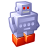# QlikView App Dev

Discussion Board for collaboration related to QlikView App Development.

Announcements
Welcome to Qlik Community! Check out our new navigation! FIND OUT MORE
cancel
Showing results for
Did you mean:Creator III

## Colour Conditioning for perecntages for in chart text

Hi Guys

I have an expression

I am trying to color code the text

My value is a percentage

Below keeps the value to green

And it does not change

This is not working:

=IF(vUT%>=0.0 and vUT% <= 0.5, RED(),

IF(vUT%>=0.51 and vUT% <= 0.7, YELLOW(),

Green()))

1 Solution

Accepted SolutionsMaster II

=IF((sum(Sales)/sum(Total Sales))>=0.0 and (sum(Sales)/sum(Total Sales)) <= 0.5, RED(),

IF((sum(Sales)/sum(Total Sales))>=0.51 and (sum(Sales)/sum(Total Sales)) <= 0.7, YELLOW(),

Green()))

10 RepliesContributor III

Hi Aaron,

Could please provide the sample value of vUT% field so that i can provide you the solution

Thanks

AnkurSpecialist

Hi

Can you plz share a sample app?

Regards

PratyushMVP

Can you use Square Brackets ([]) around your expression. What does the expression for vUT% and how values are looks like?

=IF([vUT%] >= 0.0 and [vUT%] <= 0.5, RED(),

IF([vUT%]>=0.51 and [vUT%] <= 0.7, YELLOW(),

Green()))

Before develop something, think If placed (The Right information | To the right people | At the Right time | In the Right place | With the Right context)Creator III
Author

Im sorry pratyush i cannot,

the value stays green and does not change to red or yellow depending on what it is

i.e: If the percentage is lower than 50% it should be red

higher than 70% it should be greenSpecialist

Hi

Can you just give me an idea as to how you've declared the variable?

Regards

PratyushCreator III
Author

vUT%= (avg(Aggr(sum(JO_)/(TGT),_Vehicle)))

HTHSpecialist

It is giving green coz it is not satisfying any of the conditions above, try to use the variable in text. Capture its value and then try to check that in your condition.

Regards
PratyushMaster II

=IF((sum(Sales)/sum(Total Sales))>=0.0 and (sum(Sales)/sum(Total Sales)) <= 0.5, RED(),

IF((sum(Sales)/sum(Total Sales))>=0.51 and (sum(Sales)/sum(Total Sales)) <= 0.7, YELLOW(),

Green()))Creator III
Author

Sorry could you explain more?